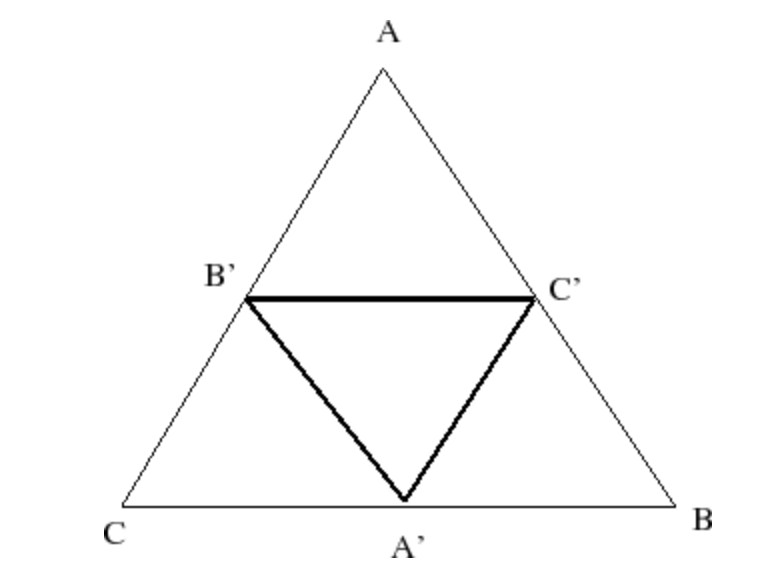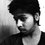# Triangle in Triangle$$Question:$$.

How to Find The minimum possible perimeter of an triangle A'B'C' which is inscribed in an another acute angled Triangle ABC ??

$SOURCE:$. Ask by a friend.

$MY WORK:$.

In my views A'B'C' must be orthic triangle ( i.e Pedal Triangle of ABC ) but I'am stuck at how to calculate minimum perimeter??

Perimeter is Given By :

Perimeter of triangle A'B'C' = R[ Sin(2A) + Sin(2B) + Sin(2C) ]

Doubts

1)- But $R=unknown$. & $Angles=unknown$. So how To convert All of them in single variables ???

2)- I'am also thinking of some reflection with respect to sides Concept (which is suggest by my friend) But not successful in it.

3)- Is it Can be solved Without using Reflection's .

Will someone Help me ????

Update

$Proof$. : Proof of inner triangle To be orthic triangle is: ( I'am not 100% Sure since it is not Pure Mathematical and it need's some concept's of Optics like as Fermat Principle ).

According to Me A'B'C' is orthic triangle because if you placed an light source at A' such that light it emits from A' traces the perimeter of Triangle A'B"C . Then For Tracing minimum perimeter it should Follow the Path of light (According To Fermat's principle) So A'B' must be Incident ray on side BC of bigger Triangle And B'C' Must be reflected ray of light.

Similarly for Side B'C' and for C'A'. Now The If we draw Normal at points A' , B' , C' then They must intersect inside The Triangle A'B'C' . Let their Point of intersection is 'H' then 'H' must be orthocenter of triangle ABC since it is intersection of Altitudes of Triangles

Since normal line HC' divides angle $\angle A'B'C'\quad$. in two equal parts i.e. $\angle A'B'H\quad =\quad \angle C'B'H$. which arise from fact that incidence angle = reflected angle ,Hence These Normal line of triangle ABC are the angle bisectors for inner triangle which Always intersect at Orthocenter of outer triangle

So if join these Foot of perpendiculars which are A' & B' & C' then they must form Pedal Triangle of bigger triangle.

$NOTE$. : Fact that i used in Proof is that :

If the triangle ABC is oblique (not right-angled), the points of intersection of the altitudes with the sides of the triangle form another triangle, A'B'C', called the orthic triangle or altitude triangle. It is the pedal triangle of the orthocenter of the original triangle. Also, the incenter (that is, the center for the inscribed circle) of the orthic triangle is the orthocenter of the original triangleNote by Deepanshu Gupta
6 years ago

This discussion board is a place to discuss our Daily Challenges and the math and science related to those challenges. Explanations are more than just a solution — they should explain the steps and thinking strategies that you used to obtain the solution. Comments should further the discussion of math and science.

When posting on Brilliant:

• Use the emojis to react to an explanation, whether you're congratulating a job well done , or just really confused .
• Ask specific questions about the challenge or the steps in somebody's explanation. Well-posed questions can add a lot to the discussion, but posting "I don't understand!" doesn't help anyone.
• Try to contribute something new to the discussion, whether it is an extension, generalization or other idea related to the challenge.

MarkdownAppears as
*italics* or _italics_ italics
**bold** or __bold__ bold
- bulleted- list
• bulleted
• list
1. numbered2. list
1. numbered
2. list
Note: you must add a full line of space before and after lists for them to show up correctly
paragraph 1paragraph 2

paragraph 1

paragraph 2

[example link](https://brilliant.org)example link
> This is a quote
This is a quote
    # I indented these lines
# 4 spaces, and now they show
# up as a code block.

print "hello world"
# I indented these lines
# 4 spaces, and now they show
# up as a code block.

print "hello world"
MathAppears as
Remember to wrap math in $$ ... $$ or $ ... $ to ensure proper formatting.
2 \times 3 $2 \times 3$
2^{34} $2^{34}$
a_{i-1} $a_{i-1}$
\frac{2}{3} $\frac{2}{3}$
\sqrt{2} $\sqrt{2}$
\sum_{i=1}^3 $\sum_{i=1}^3$
\sin \theta $\sin \theta$
\boxed{123} $\boxed{123}$

Sort by:

hmm....Your Proof seems To be logical..!!

- 6 years ago

you can refer the blog "rgpkendra.blogspot.in" for proof. Its easy using reflections.

- 6 years ago

- 6 years ago

Is it has similiar concept of your question which is Previously posted by you of named "DATA INSUFFICIENT.....???"

- 6 years ago

Actually I created That Question From This Question....!!

- 6 years ago

This is actually a very famous problem. But the problem is to prove that minimum possible perimeter is of orthic triangle. This is actually a beautiful result, and its extremely hard to prove. How did you proved the result?. This is commonly known as fragnano's problem. I read about it when i was preparing for olympiads in geometry revisited and it has very beautiful solution using reflections repeatedly. you can study about it , from http://www.cut-the-knot.org/triangle/Fagnano.shtml .

And what do you mean by what is done for 'R'?. You can obviously write it in terms of $a$ and $A$ . After all it will be dependent on sides the triangle.

- 6 years ago

Correct me if I'am wrong..!!

According to Me A'B'C' is orthic triangle because if you placed an light source at A' such that light it emits from A' traces the perimeter of Triangle A'B"C . Then For Tracing minimum perimeter it should Follow the Path of light (According To Fermat's principle) So A'B' must be Incident ray on side BC of bigger Triangle And B'C' Must be reflected ray of light.

Similarly for Side B'C' and for C'A'. Now The If we draw Normal at points A' , B' , C' then They must intersect inside The Triangle A'B'C' . Let their Point of intersection is 'H' then 'H' must be orthocenter of triangle ABC since it is intersection of Altitudes of Triangles

(Since normal line HC' divides angle $\angle A'B'C'\quad$. in two equal parts i.e. $\angle A'B'H\quad =\quad \angle C'B'H$. which arise from fact that incidence angle = reflected angle ,Hence These Normal line of triangle ABC are the angle bisectors for inner triangle which Always intersect at Orthocenter of outer triangle)

. So if join these Foot of perpendiculars which are A' & B' & C' then they must form Pedal Triangle of bigger triangle.

And Also i want to say that if we Fixed R then Maximum value of Perimeter is : $P=\frac { 3\sqrt { 3 } }{ 2 } R$. So previously I have confusion that is this question is complete or not but now (as you told that it is Very famous Question ) i feel that this question is obviously correct.

But how can this question is solved without using Reflections....??

- 6 years ago